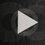# Proof WritingI often see people who have a solution, and go like "Wow! I proved it!". A solution is a completely different thing than a proof. Here are the definitions of the two from Wolfram Alpha:

Solution | noun | a method for solving a problem.

Proof | noun | a rigorous mathematical argument which unequivocally demonstrates the truth of a given proposition.

See the difference? You didn't prove it, you just showed a solution. Now, I will show you some steps for proof writing.

Step 1: Figure out what you are asked. If the problem said how many steps Jack took to run a mile, you would be looking for the number of steps Jack took. Always state what you are looking for at the beginning of any proof.

Step 2: Prove what you are looking for. There are multiple ways to do this, but I would always recommend a paragraph proof. One type of paragraph proof is induction. This works well when trying to prove an equation. You first prove the equation works for $1$. Then you assume the equation works for $x$. Then you prove it works for $x+1$. If you prove it works for all of these, the equation is proved. There are many other ways to prove things that I won't go into, but you can find your own ways or look them up.

Step 3: Say what [Step 2] means in terms of the problem. If you use induction, and prove it for those three, so what? How will the persons reading it know what you mean? You need to state what it mean in terms of the problem. If you prove that $x^{5}=y^{3}$, what does that mean in the problem?

Step 4: Summarize. You have just proven and explained what everything is. Now it is time to wrap it up. Summarize what you did [Step 2] and write the answer clearly and boxed. Some people might start from the end and go to the front, so write this part clearly. And remember, always end with a QED or an $\boxed { }$, to show that you are done.

Well, now you know the basics of writing a proof. If you feel I'm missing something, or you just want to say thanks, comment! I'm open to suggestions!Note by Chris Hambacher
7 years, 2 months ago

This discussion board is a place to discuss our Daily Challenges and the math and science related to those challenges. Explanations are more than just a solution — they should explain the steps and thinking strategies that you used to obtain the solution. Comments should further the discussion of math and science.

When posting on Brilliant:

• Use the emojis to react to an explanation, whether you're congratulating a job well done , or just really confused .
• Ask specific questions about the challenge or the steps in somebody's explanation. Well-posed questions can add a lot to the discussion, but posting "I don't understand!" doesn't help anyone.
• Try to contribute something new to the discussion, whether it is an extension, generalization or other idea related to the challenge.

MarkdownAppears as
*italics* or _italics_ italics
**bold** or __bold__ bold
- bulleted- list
• bulleted
• list
1. numbered2. list
1. numbered
2. list
Note: you must add a full line of space before and after lists for them to show up correctly
paragraph 1paragraph 2

paragraph 1

paragraph 2

[example link](https://brilliant.org)example link
> This is a quote
This is a quote
    # I indented these lines
# 4 spaces, and now they show
# up as a code block.

print "hello world"
# I indented these lines
# 4 spaces, and now they show
# up as a code block.

print "hello world"
MathAppears as
Remember to wrap math in $$ ... $$ or $ ... $ to ensure proper formatting.
2 \times 3 $2 \times 3$
2^{34} $2^{34}$
a_{i-1} $a_{i-1}$
\frac{2}{3} $\frac{2}{3}$
\sqrt{2} $\sqrt{2}$
\sum_{i=1}^3 $\sum_{i=1}^3$
\sin \theta $\sin \theta$
\boxed{123} $\boxed{123}$

Sort by:

Nice introductory post! One or two examples would have made the post even better.

Correction: When you prove something inductively, you don't prove that it works for $x$. You assume that it works for $x$ and you show that if it works for $k$, it works for $k+1$ as well.

For example, if you have a statement such that if it works for $k$, it works for $k+1$; and then show that it works for $7$, you prove that the statement works for any positive integer $\geq 7$.

- 7 years, 2 months ago

Sorry 'bout that!

- 7 years, 2 months ago

There's nothing to be sorry about! You can edit your note. Changing 'prove' into 'assume' in step 2 would do.

- 7 years, 2 months ago

Done!

- 7 years, 2 months ago

Nice! It will help me a lot! Thanx!

- 7 years, 2 months ago

Hey kunal ...how much did you get in JEE mains

- 7 years, 2 months ago

and also other exams like BTSAT etc.

- 7 years, 2 months ago